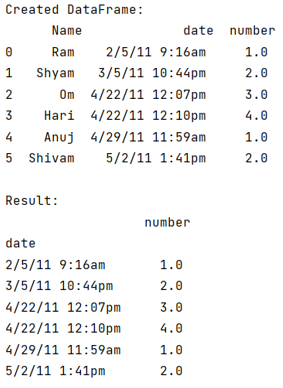# Pandas DataFrame groupby datetime month

Given a Pandas DataFrame, we have to groupby datetime month. By Pranit Sharma Last updated : September 23, 2023

Pandas is a special tool that allows us to perform complex manipulations of data effectively and efficiently. Inside pandas, we mainly deal with a dataset in the form of DataFrame. DataFrames are 2-dimensional data structures in pandas. DataFrames consists of rows, columns, and the data. The Data inside the DataFrame can be of any type.

Usually, DataFrames are created with the help of dictionaries but we can also create a DataFrame with the help of strings.

## Problem statement

Given a Pandas DataFrame, we have to groupby datetime month.

## DataFrame groupby datetime month

Here, we have a date and time column in a string which we will convert into a DataFrame, then we will group by datetime and we will observe the count of each value, for this purpose, we will use groupby() method and apply sum() method to it.

Note

To work with pandas, we need to import pandas package first, below is the syntax:

```import pandas as pd
```

Let us understand with the help of an example,

## Python Program for Pandas DataFrame groupby datetime month

```# Importing pandas package
import pandas as pd

# Importing StringIO module from io module
from io import StringIO

# Creating a string
string= StringIO("""
Name,date,number
Ram,2/5/11 9:16am,1.0
Shyam,3/5/11 10:44pm,2.0
Om,4/22/11 12:07pm,3.0
Hari,4/22/11 12:10pm,4.0
Anuj,4/29/11 11:59am,1.0
Shivam,5/2/11 1:41pm,2.0
""")

# Reading String in form of csv file

# Convert df into dataframe
df = pd.DataFrame(df)

# Display dataframe
print("Created DataFrame:\n",df,"\n")

# Groupby datetime
result = df.groupby('date').sum()

# Display result
print("Result:\n",result)
```

### Output

The output of the above program is: